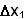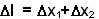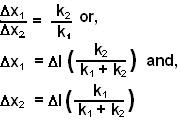Friday, May 2, 2008

Irodov Problem 1.129Letandbe the extensions in the two springs. The total extension of both the springs combined together is. Let F be the force which is being applied to stretch the springs.

The most important thing is to notice is that both the springs will experience the same tension. Why? Suppose that the tension in spring 1 was F1 then spring 2 would experience a force F at one end while F1 at the other end. This would mean that there would be a net force of F-F1 acting on the spring. But this cannot be true since the springs are not accelerating, they are simply stretched!! This means F-F1=0 or F=F1. In other words tension in both springs must be the same. This actually holds for any number of springs connected in series.

Hence we have,In other words,The work done by this force will be entirely the energy stored in the springs given by,